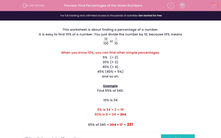# Find Percentages of the Given Numbers

In this worksheet, students find a percentage of a given number.Key stage:  KS 2

Curriculum topic:   Maths and Numerical Reasoning

Curriculum subtopic:   Percentages

Difficulty level:#### Worksheet Overview

This worksheet is about finding a percentage of a number.

It is easy to find 10% of a number. You just divide the number by 10, because 10% means

 10 100
or
 1 10

When you know 10%, you can find other simple percentages.

5%   (÷ 2)

20% (× 2)

40% (× 4)

45% (40% + 5%)

and so on.

Example

Find 65% of 340.

10% is 34

5% is 34 ÷ 2 = 17

60% is 6 × 34 = 204

65% of 340 = 204 + 17 = 221

### What is EdPlace?

We're your National Curriculum aligned online education content provider helping each child succeed in English, maths and science from year 1 to GCSE. With an EdPlace account you’ll be able to track and measure progress, helping each child achieve their best. We build confidence and attainment by personalising each child’s learning at a level that suits them.

Get started